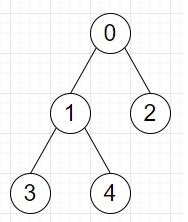# Program to count number of unique paths that includes given edges in Python

Suppose we have a list of edges in the form (u, v) and these are representing a tree. For each edge we have to find the total number of unique paths that includes said edge, in the same order as given in the input.

So, if the input is like edges = [[0, 1],[0, 2],[1, 3],[1, 4]]then the output will be [6, 4, 4, 4].

To solve this, we will follow these steps −

• count := an empty map

• Define a function dfs() . This will take x, parent

• count[x] := 1

• for each nb in adj[x], do

• if nb is same as parent, then

• come out from the loop

• count[x] := count[x] + dfs(nb, x)

• return count[x]

• From the main method do the following −

• dfs(0, -1)

• ans := a new list

• for each edge (a, b) in edges, do

• x := minimum of count[a] and count[b]

• insert (x *(count - x)) at the end of ans

• return ans

## Example

Let us see the following implementation to get better understanding −

Live Demo

from collections import defaultdict
class Solution:
def solve(self, edges):
for a, b in edges:
count = defaultdict(int)
def dfs(x, parent):
count[x] = 1
if nb == parent:
continue
count[x] += dfs(nb, x)
return count[x]
dfs(0, -1)
ans = []
for a, b in edges:
x = min(count[a], count[b])
ans.append(x * (count - x))
return ans
ob = Solution()
edges = [
[0, 1],
[0, 2],
[1, 3],
[1, 4]
]
print(ob.solve(edges))

## Input

[
[0, 1],
[0, 2],
[1, 3],
[1, 4]
]

## Output

[6, 4, 4, 4]